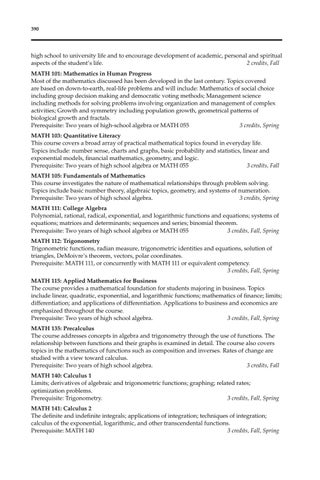390

high school to university life and to encourage development of academic, personal and spiritual aspects of the studentâ&#x20AC;&#x2122;s life. 2 credits, Fall MATH 101: Mathematics in Human Progress Most of the mathematics discussed has been developed in the last century. Topics covered are based on down-to-earth, real-life problems and will include: Mathematics of social choice including group decision making and democratic voting methods; Management science including methods for solving problems involving organization and management of complex activities; Growth and symmetry including population growth, geometrical patterns of biological growth and fractals. Prerequisite: Two years of high-school algebra or MATH 055 3 credits, Spring MATH 103: Quantitative Literacy This course covers a broad array of practical mathematical topics found in everyday life. Topics include: number sense, charts and graphs, basic probability and statistics, linear and exponential models, financial mathematics, geometry, and logic. Prerequisite: Two years of high school algebra or MATH 055 3 credits, Fall MATH 105: Fundamentals of Mathematics This course investigates the nature of mathematical relationships through problem solving. Topics include basic number theory, algebraic topics, geometry, and systems of numeration. Prerequisite: Two years of high school algebra. 3 credits, Spring MATH 111: College Algebra Polynomial, rational, radical, exponential, and logarithmic functions and equations; systems of equations; matrices and determinants; sequences and series; binomial theorem. Prerequisite: Two years of high school algebra or MATH 055 3 credits, Fall, Spring MATH 112: Trigonometry Trigonometric functions, radian measure, trigonometric identities and equations, solution of triangles, DeMoivreâ&#x20AC;&#x2122;s theorem, vectors, polar coordinates. Prerequisite: MATH 111, or concurrently with MATH 111 or equivalent competency. 3 credits, Fall, Spring MATH 115: Applied Mathematics for Business The course provides a mathematical foundation for students majoring in business. Topics include linear, quadratic, exponential, and logarithmic functions; mathematics of finance; limits; differentiation; and applications of differentiation. Applications to business and economics are emphasized throughout the course. Prerequisite: Two years of high school algebra. 3 credits, Fall, Spring MATH 135: Precalculus The course addresses concepts in algebra and trigonometry through the use of functions. The relationship between functions and their graphs is examined in detail. The course also covers topics in the mathematics of functions such as composition and inverses. Rates of change are studied with a view toward calculus. Prerequisite: Two years of high school algebra. 3 credits, Fall MATH 140: Calculus 1 Limits; derivatives of algebraic and trigonometric functions; graphing; related rates; optimization problems. Prerequisite: Trigonometry. 3 credits, Fall, Spring MATH 141: Calculus 2 The definite and indefinite integrals; applications of integration; techniques of integration; calculus of the exponential, logarithmic, and other transcendental functions. Prerequisite: MATH 140 3 credits, Fall, Spring